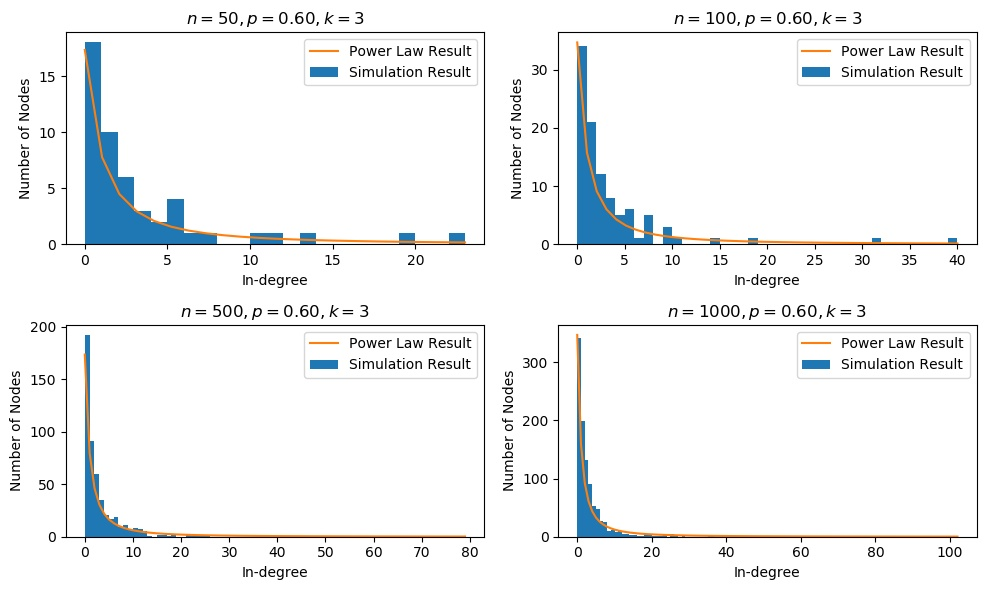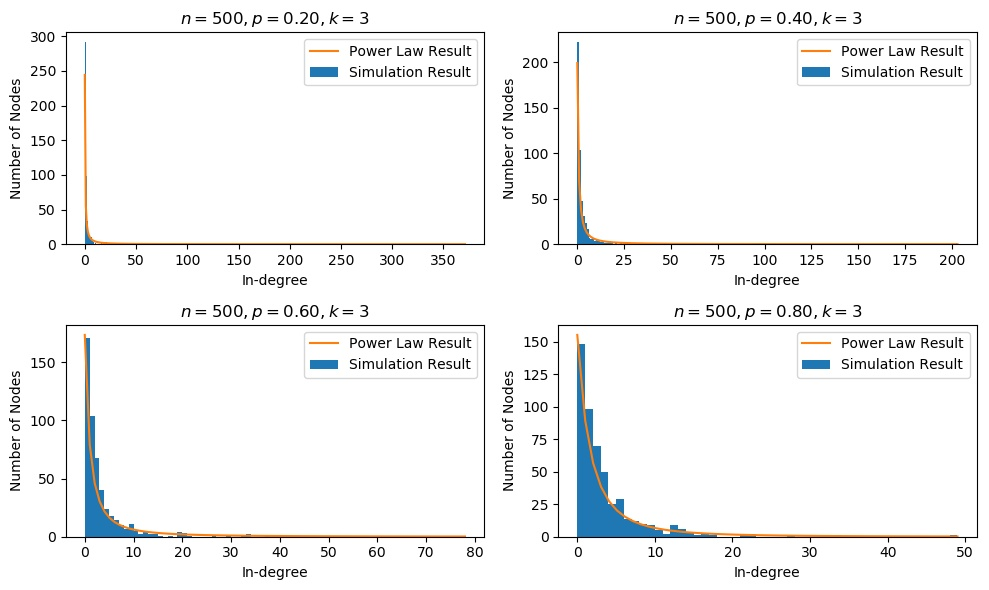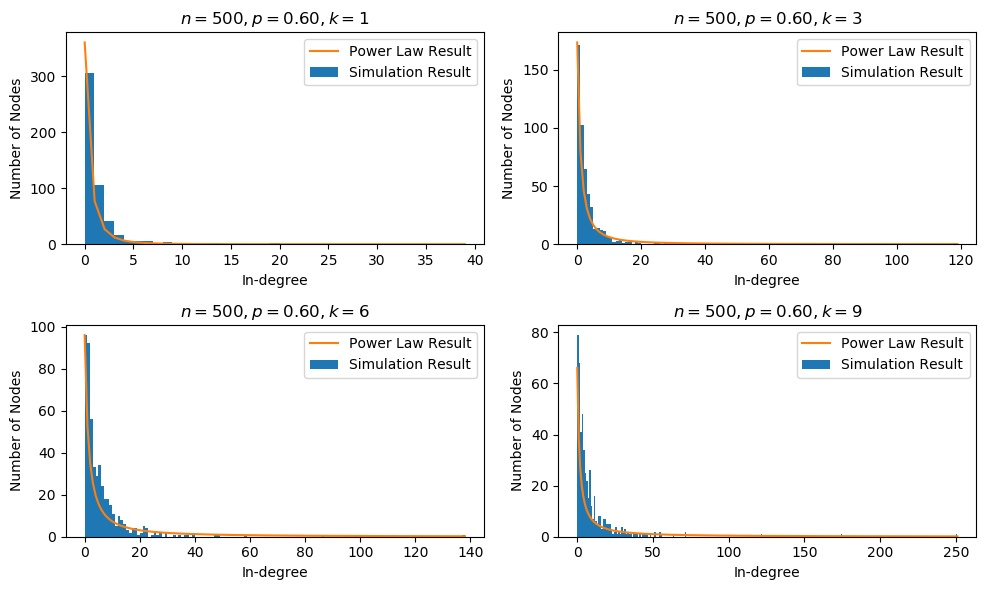## 数学模型

(a) 在已有的 $j-1$ 个节点中均匀地, 随机地选择一个早先创建的网页 $i$, 建立一个从 $j$ 到 $i$ 的链接. 选中每一个节点的概率为 $1/(j-1)$.
(b) 按照与已有入度成比例的概率, 选择一个早先创建的网页 $i$，建立一个从 $j$ 到 $i$ 的链接. 选中每一个节点的概率与该节点的现有入度成正比.

## 实验结果

### 改变 $n$ 的实验### 改变 $p$ 的实验### 改变 $k$ 的实验Welcome to my other publishing channels